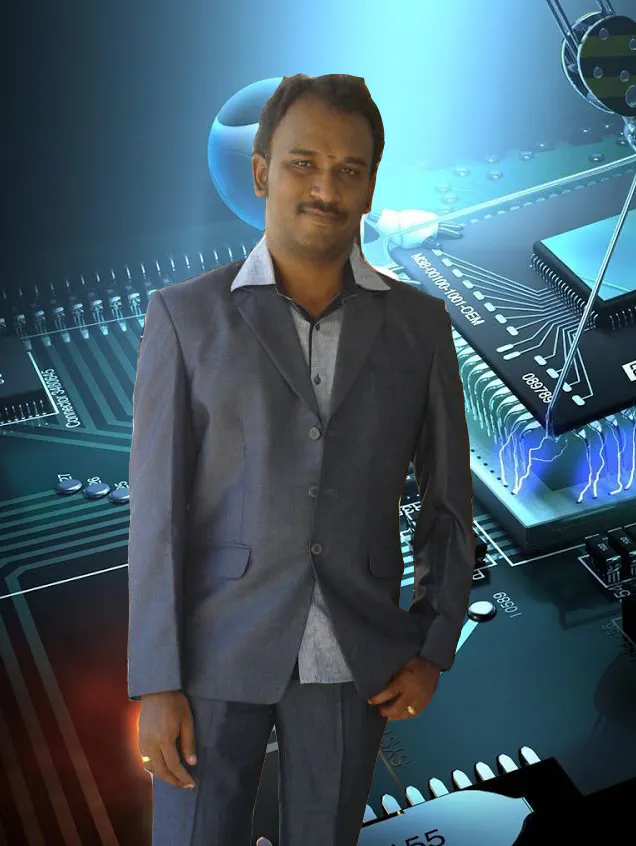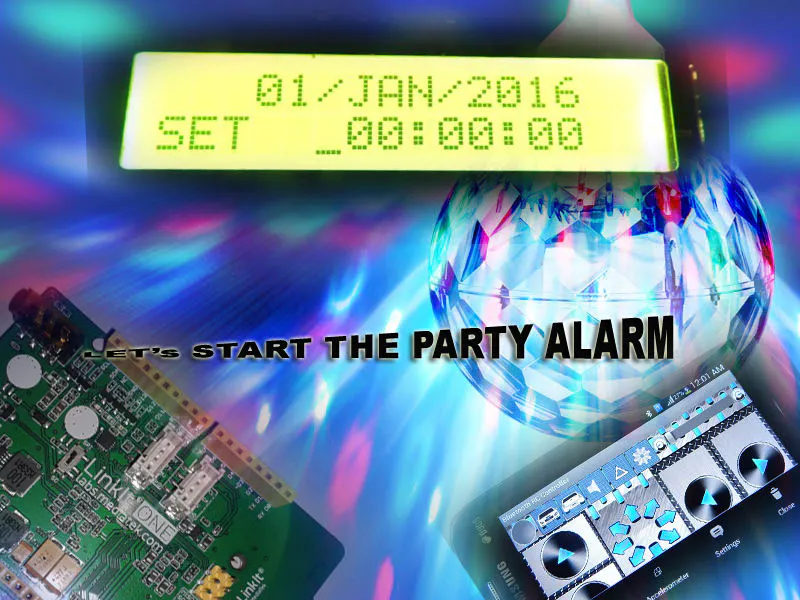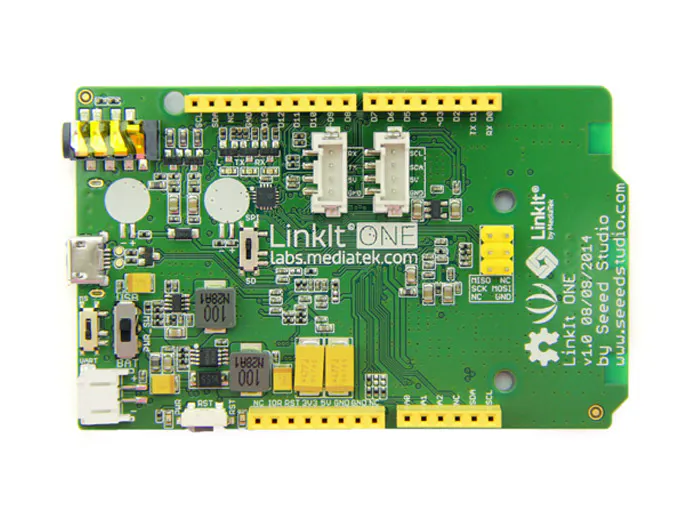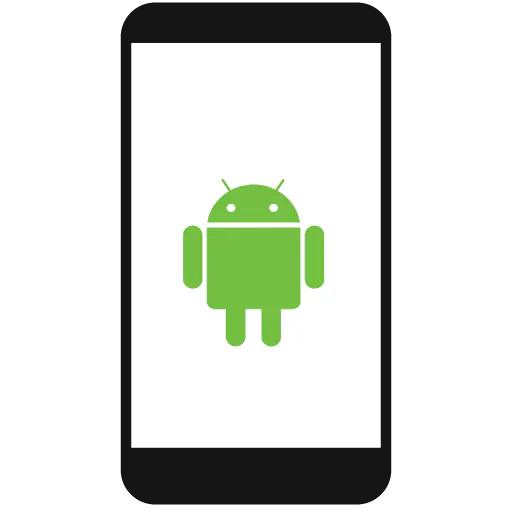# Light and Music Party Start Alarm using BT Settings

Start the party at correct time with out delay.

IntermediateFull instructions provided224## Things used in this project

### Hardware components×1
 Serial 16X2 LCD display
×1
 speakers
×1
 Party Lights
×1
 Push Button
×1
 Relay (generic)
×1Android device
×1

### Software apps and online services

 Bluetooth RC car App

### Hand tools and fabrication machinesSoldering iron (generic)

## Code

### Partystart.ino

Arduino
```#include <LAudio.h>
#include <LBT.h>
#include <LBTServer.h>
#include <LDateTime.h>
#include <LEEPROM.h>

datetimeInfo t;
unsigned int rtc;
unsigned int Lrtc;

String Cfilename;
int start=0;
AudioStatus status1;

int cmonth=0;
int cday=0;
int cyear=0;
int chour=0;
int cmin=0;
int csec=0;

int smonth=0;
int sday=0;
int syear=0;
int shour=0;
int smin=0;
int ssec=0;

char keydown;

int changein=0;
int changeid=0;

String Display1;
String Display2;

String getmon;
int sumday;

boolean alaram=false;
int buttondown=0;
int buttonno=A1;
int buttonval=0;

int relayno=13;

void setup() {
if(!LBTServer.begin((uint8_t*)"Sivam_LIO"))
{
return;
}
//  Serial.begin(115200);
Serial1.begin(9600);
LDateTime.getTime(&t);
if (t.year<2015)
{
t.mon=12;
t.day=14;
t.year=2015;
t.hour=16;
t.min=12;
t.sec=36;
LDateTime.setTime(&t);
}
pinMode(relayno, OUTPUT);
{
smonth=7;
syear=1984;
sday=8;
shour=12;
smin=0;
ssec=0;
}
else
{
}

Serial1.write(24);
Serial1.write(17);
lcddisplay();
}

void lcddisplay()
{
if (changein==0)
{
Display1="   ";
if (cday<10)
{
Display1=Display1+"0";
}
Display1=Display1+cday;
Display1=Display1+"/";
fmonth(cmonth);
Display1=Display1+getmon;
Display1=Display1+"/";
Display1=Display1+cyear;
Display1=Display1+"  ";

Display2="CUR   ";
if (chour<10)
{
Display2=Display2+"0";
}
Display2=Display2+chour;
Display2=Display2+":";
if (cmin<10)
{
Display2=Display2+"0";
}
Display2=Display2+cmin;
Display2=Display2+":";
if (csec<10)
{
Display2=Display2+"0";
}
Display2=Display2+csec;
Display2=Display2+"  ";

Serial1.write(128);
Serial1.println(Display1);
Serial1.write(148);
Serial1.println(Display2);
} else
{
Display1="   ";
if (sday<10)
{
Display1=Display1+"0";
}
Display1=Display1+sday;
Display1=Display1+"/";
fmonth(smonth);
Display1=Display1+getmon;
Display1=Display1+"/";
Display1=Display1+syear;
Display1=Display1+"  ";

Display2="SET   ";
if (shour<10)
{
Display2=Display2+"0";
}
Display2=Display2+shour;
Display2=Display2+":";
if (smin<10)
{
Display2=Display2+"0";
}
Display2=Display2+smin;
Display2=Display2+":";
if (ssec<10)
{
Display2=Display2+"0";
}
Display2=Display2+ssec;
Display2=Display2+"  ";
Serial1.write(128);
Serial1.println(Display1);
Serial1.write(148);
Serial1.println(Display2);
}

if(changeid==1)
{
Serial1.write(131);
}else if(changeid==2)
{
Serial1.write(134);
}else if(changeid==3)
{
Serial1.write(138);
}else if(changeid==4)
{
Serial1.write(154);
}else if(changeid==5)
{
Serial1.write(157);
}else if(changeid==6)
{
Serial1.write(160);
}
}

void fmonth(int mon)
{
if (mon==1)
getmon="JAN";
else if (mon==2)
getmon="FEB";
else if (mon==3)
getmon="MAR";
else if (mon==4)
getmon="APR";
else if (mon==5)
getmon="MAY";
else if (mon==6)
getmon="JUN";
else if (mon==7)
getmon="JUL";
else if (mon==8)
getmon="AUG";
else if (mon==9)
getmon="SEP";
else if (mon==10)
getmon="OCT";
else if (mon==11)
getmon="NOV";
else if (mon==12)
getmon="DEC";
}

void buttonpress()
{
if (buttonval>100 && buttondown==0)
{
buttondown=1;
}else if (buttonval<100 && buttondown==1)
{
buttondown=0;
if(alaram==true)
{
alaram=false;
LAudio.stop();
}else if (changein==0)
{
changein=1;
}else if (changein==1)
{
changein=0;
}
}
}

void loop() {
uint8_t buf;
if(LBTServer.connected())
{
buttonpress();
while(true)
{
buttonpress();
break;
if (char(readval)!='S' and keydown == 'S')
{
}else if (char(readval)=='S' and keydown != 'S')
{
if (keydown=='L' || keydown=='R')
{
}else if (keydown=='F' || keydown=='B')
{
if(changein==1)
changeset(keydown);
else
changecur(keydown);
}
keydown='S';
}
printdatetime();
}
printdatetime();
delay(100);
}
else
{
buttonpress();
changeid=1;
printdatetime();
LBTServer.accept(1);
}
}

void saveset()
{
int extyear1;
int extyear2;
extyear2=syear%100;
extyear1=(syear-extyear2)/100;
}

{
{
if (changeid==1)
{
sday=sumday;
} else if (changeid==2)
{
smonth=smonth+1;
if (smonth>12)
{
smonth=1;
}
}else if (changeid==3)
{
syear=syear+1;
if(syear>9999)
{
syear=1000;
}
}else if (changeid==4)
{
shour=shour+1;
if (shour>23)
{
shour=0;
}
}else if (changeid==5)
{
smin=smin+1;
if (smin>59)
{
smin=0;
}
}else if (changeid==6)
{
ssec=ssec+1;
if (ssec>59)
{
ssec=0;
}
}
saveset();
}
{
if (changeid==1)
{
daysub(sday,smonth,syear);
sday=sumday;
} else if (changeid==2)
{
smonth=smonth-1;
if (smonth<1)
{
smonth=12;
}
}else if (changeid==3)
{
syear=syear-1;
if(syear<1000)
{
syear=9999;
}
}else if (changeid==4)
{
shour=shour-1;
if (shour<0)
{
shour=23;
}
}else if (changeid==5)
{
smin=smin-1;
if (smin<0)
{
smin=59;
}
}else if (changeid==6)
{
ssec=ssec-1;
if (ssec<0)
{
ssec=59;
}
}
saveset();
}
}

void savecur()
{
t.mon=cmonth;
t.day=cday;
t.year=cyear;
t.hour=chour;
t.min=cmin;
t.sec=csec;
LDateTime.setTime(&t);
}
{
{
if (changeid==1)
{
cday=sumday;
} else if (changeid==2)
{
cmonth=cmonth+1;
if (cmonth>12)
{
cmonth=1;
}
}else if (changeid==3)
{
cyear=cyear+1;
if(cyear>9999)
{
cyear=1000;
}
}else if (changeid==4)
{
chour=chour+1;
if (chour>23)
{
chour=0;
}
}else if (changeid==5)
{
cmin=cmin+1;
if (cmin>59)
{
cmin=0;
}
}else if (changeid==6)
{
csec=csec+1;
if (csec>59)
{
csec=0;
}
}
savecur();
}
{
if (changeid==1)
{
daysub(cday,cmonth,cyear);
cday=sumday;
} else if (changeid==2)
{
cmonth=cmonth-1;
if (cmonth<1)
{
cmonth=12;
}
}else if (changeid==3)
{
cyear=cyear-1;
if(cyear<1000)
{
cyear=9999;
}
}else if (changeid==4)
{
chour=chour-1;
if (chour<0)
{
chour=23;
}
}else if (changeid==5)
{
cmin=cmin-1;
if (cmin<0)
{
cmin=59;
}
}else if (changeid==6)
{
csec=csec-1;
if (csec<0)
{
csec=59;
}
}
savecur();
}
}

{
curday = curday+1;
int leapyear;
if (curmon==1 || curmon==3 || curmon==5 || curmon==7 || curmon==8 || curmon==10 || curmon==12)
{
if (curday>31)
{
curday=1;
}
}else if (curmon==4 || curmon==6 || curmon==9 || curmon==11 )
{
if (curday>30)
{
curday=1;
}
}else if (curmon==2)
{
leapyear=curyear%4;
if (leapyear==0)
{
if (curday>29)
{
curday=1;
}
}else
{
if (curday>28)
{
curday=1;
}
}
}

sumday=curday;
}

void daysub(int curday,int curmon,int curyear)
{
curday = curday-1;
int leapyear;
if (curmon==1 || curmon==3 || curmon==5 || curmon==7 || curmon==8 || curmon==10 || curmon==12)
{
if (curday<1)
{
curday=31;
}
}else if (curmon==4 || curmon==6 || curmon==9 || curmon==11 )
{
if (curday<1)
{
curday=30;
}
}else if (curmon==2)
{
leapyear=curyear%4;
if (leapyear==0)
{
if (curday<1)
{
curday=29;
}
}else
{
if (curday<1)
{
curday=28;
}
}
}

sumday=curday;
}

{
{
changeid=changeid-1;
{
changeid=changeid+1;
}

if (changeid>6)
{
changeid=1;
}else if (changeid<1)
{
changeid=6;
}
}

void printdatetime()
{
LDateTime.getTime(&t);
LDateTime.getRtc(&rtc);
if (Lrtc!=rtc)
{
Lrtc=rtc;
cmonth=t.mon;
cday=t.day;
cyear=t.year;
chour=t.hour;
cmin=t.min;
csec=t.sec;
lcddisplay();
if (alaram==true)
{
status1 = LAudio.getStatus();
if(status1 == 5 || status1 == -1)
{
play();
}
digitalWrite(relayno, HIGH);
}else
{
digitalWrite(relayno, LOW);
}
if(cday==sday && cmonth==smonth && cyear==syear)
{
if(chour==shour && cmin==smin && csec==ssec)
{
alaram=true;
digitalWrite(relayno, HIGH);
play();
delay(2000);
}
}
}
}

void play()
{
Cfilename = "Song.mp3";
char a[Cfilename.length()];
Cfilename.toCharArray(a,Cfilename.length()+1);
char *file_name;
file_name = a;
LAudio.setVolume(5);
//LAudio.playFile(storageSD, file_name);
LAudio.playFile(storageFlash, file_name);
}
```

## Credits

### jegatheesan

12 projects • 43 followers
Simply A Mechatronics Lover.